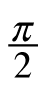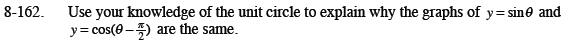Home > CCA2 > Chapter 8 > Lesson 8.3.2 > Problem8-162

8-162.

Use your knowledge of the unit circle to explain why the graphs of y = sinθ and y = cos(θ) are the same. Homework Help ✎Sketch a unit circle. Choose any angle. Find the sine and cosine of the angle. Now subtract 90° from your angle and find the sine and cosine again. What happened? If you draw an accurate reference triangle, it will help you see what is going on here.